# 使用电子表格建立交易策略

3 三月 2021, 09:19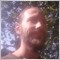0
1 034

### 快速浏览表格 - 面向初学者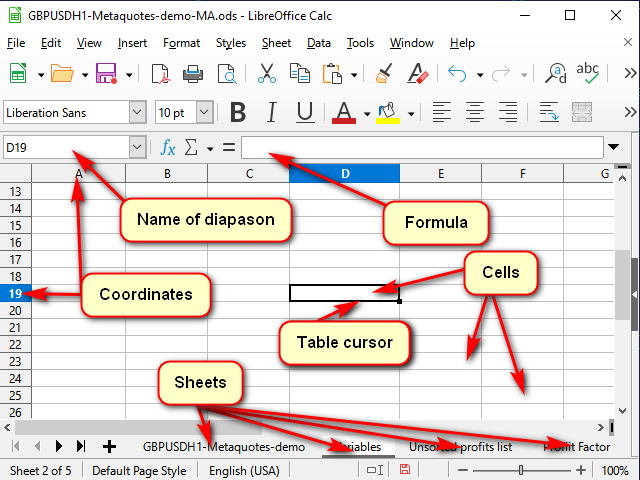ESC 键（键盘左上角）可以取消编辑而不保存。按回车(Enter)键（光标下移）或制表(Tab)键（光标移到一边），确认编辑。

### 一些使用表格的快捷键

Ctrl + 箭头

Tab 确认输入并转到右边的单元格
Shift + Tab

Enter 确认输入并转到下方的单元格
Shift + Enter

Ctrl + D

Shift + Ctrl + 箭头

### 如何用相同的公式填充长列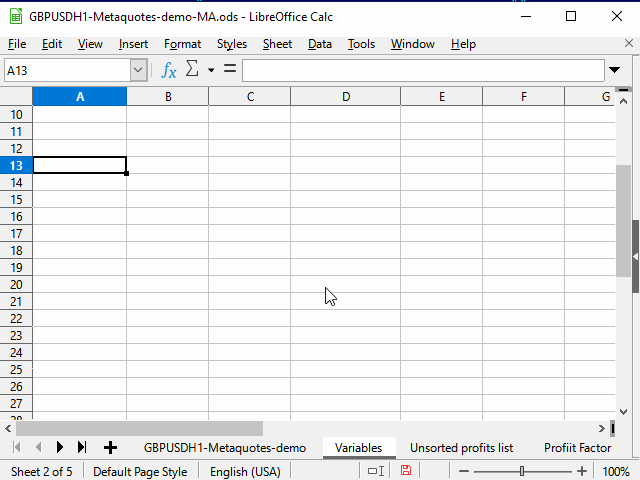#### 方法 1. 限制范围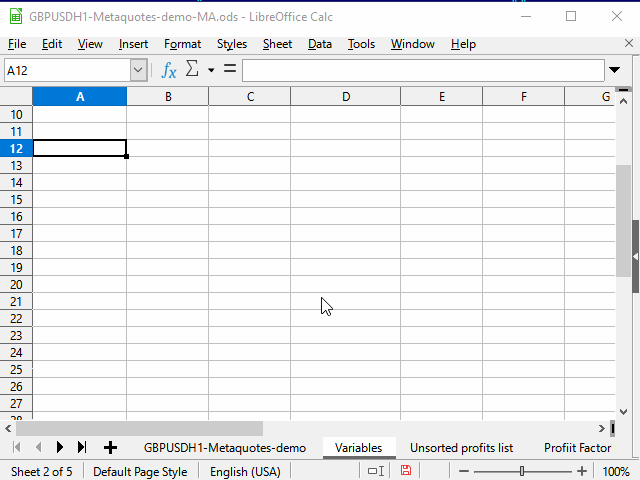1. 在范围的顶部单元格中输入所需的公式并确认输入。
2. 使用“名称”字段移到区域的最底部单元格。
3. 按下 Ctrl + Shift + 向上箭头移动到区域中最上面的单元格并选择所有中间单元格。
4. 按下 Ctrl + D 来在单元格中填充数据。

#### 方法 2. 使用相邻连续范围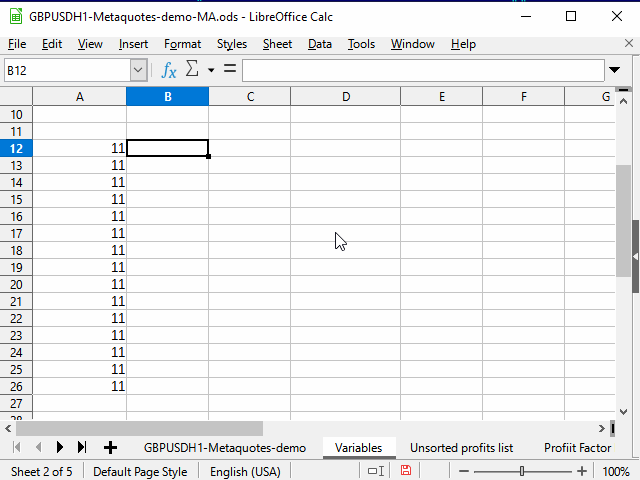1. 用必要的公式选择单元格。
2. 按下 Shift + 向左箭头来选择邻近的单元格，
3. 按下Tab来把表格光标移动到左侧单元格，在这里，我们使用表格光标的功能来仅在应用的选择中移动。
4. Ctrl + Shift + 向下箭头 — 选择连续范围内最低行的两列。
5. Shift + 向右箭头 — 取消选择左侧列，右侧列保持选中状态。
6. Ctrl + D — 使用数据填充列。

### 策略

1. 当时没有仓位并且
2. 烛形与其主体穿过移动平均线（在МА的一侧打开，在另一侧关闭）

1. 有未平仓的仓位并且
2. 烛形在与开口相反的方向穿过MA。

### 添加指标数据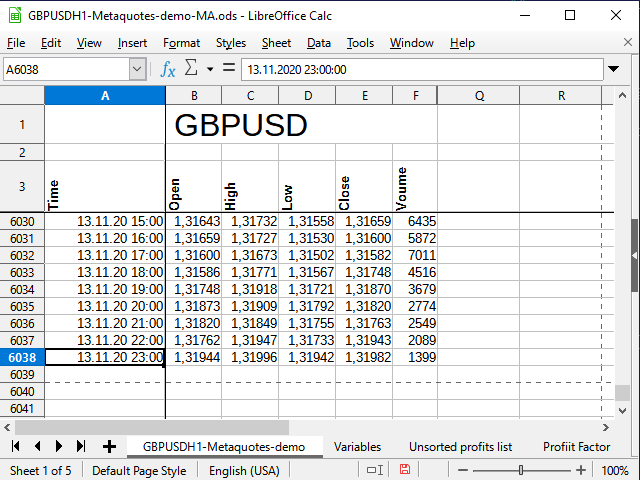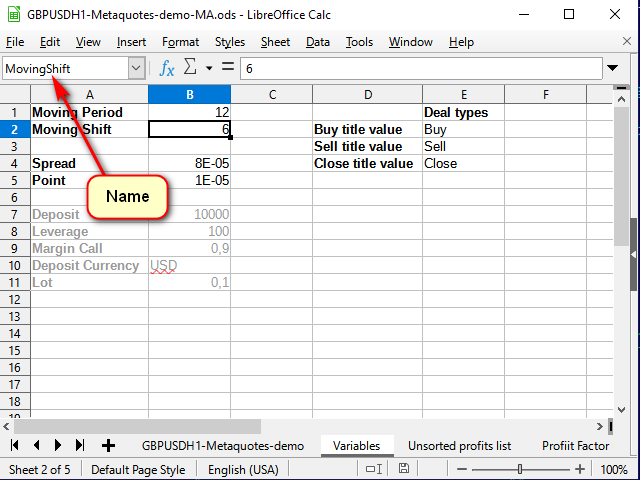`=ROW()-3`

SMA计算公式本身写在主数据表的H4中，如下所示：

`=IF( G4>(MovingPeriod+MovingShift), AVERAGE( INDIRECT( "E" & ( ROW()-MovingShift-MovingPeriod) & ":" & "E" & ( ROW()-MovingShift) ) ), "" )`

IF函数接受三个参数：条件IF 条件为 true 的值 IF 条件为false的值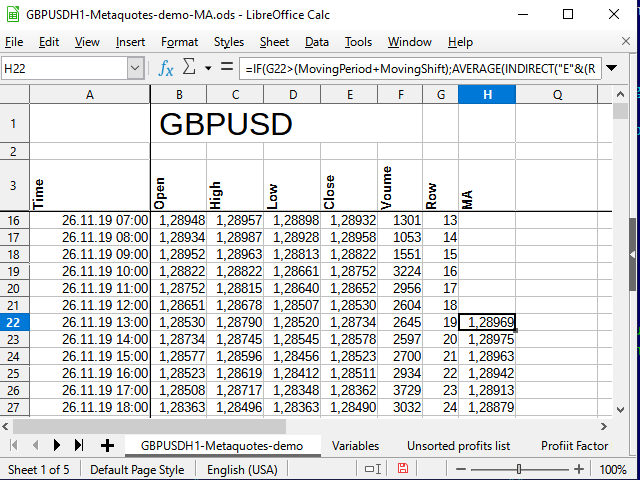### 实施策略

`=IF( AND( B4>H4,E4<H4 ),-1 , IF( AND( B4<H4,E4>H4 ), 1 , "") )`

J 这一列很适合。J4单元格中的公式如下：

`=IF(AND(I4=-1,J3=""), -1 ,IF(AND(I4=1,J3=""), 1 ,IF(OR(AND(I4="",J3=1),AND(I4="",J3=-1),I4=J3), J3 ,"")))`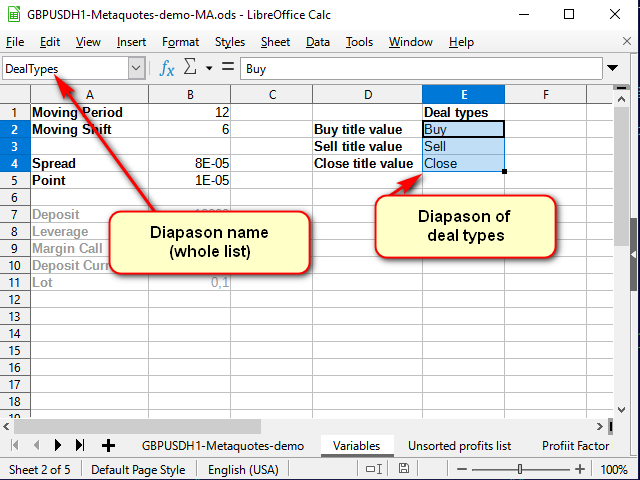`=IF(AND(J3=1,J2=""),INDEX(DealTypes,1),IF(AND(J3=-1,J2=""),INDEX(DealTypes,2),IF(OR(AND(J3="",J2=1),AND(J3="",J2=-1)),INDEX(DealTypes,3),"")))`

Index函数接受要在其中执行搜索的范围作为第一个参数。在我们的例子中，它是由名称设置的。第二个参数是范围内的行的索引。如果范围由多个列组成，要设置必要的列。如果指定了几个用分号分隔的范围，要设置从1开始的范围索引（分别是第三个和第四个参数）。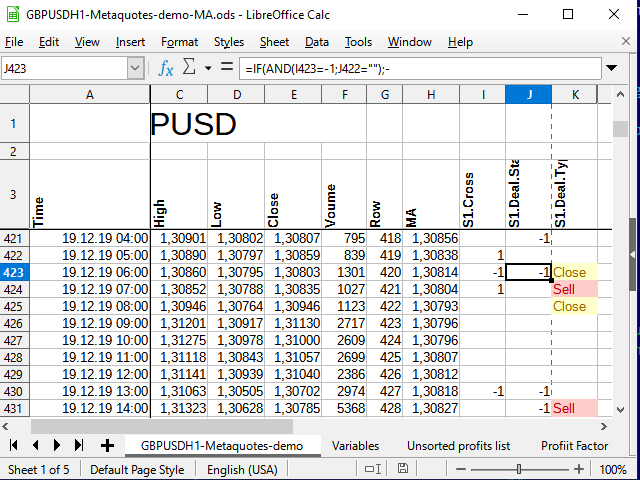### 分析策略

`=IF(K4=INDEX(DealTypes;1);B4+Spread;IF(K4=INDEX(DealTypes;2); B4 ;IF(OR(K4=INDEX(DealTypes;3);N(L3)=0); "" ;L3)))`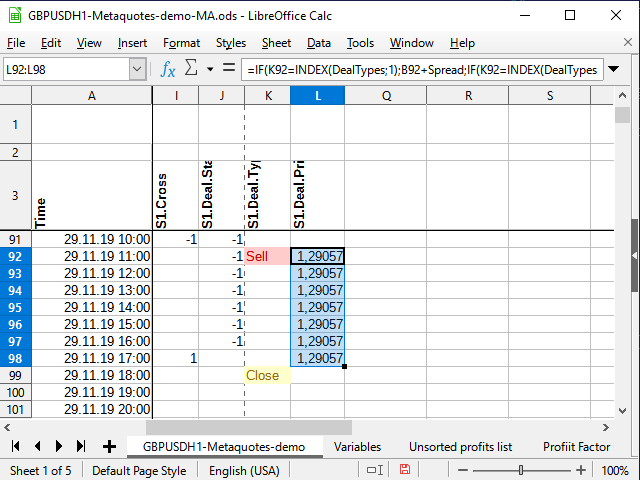`=IF(K4=INDEX(DealTypes;3);IF(I3=-1;ROUND((B4-L3)/Point);ROUND((L3-B4)/Point)); "" )`

`=IF(N4<>"";IF(COUNTIF(N\$3:N4;N4)=1;MAX(M3:M\$4)+1;"");"")`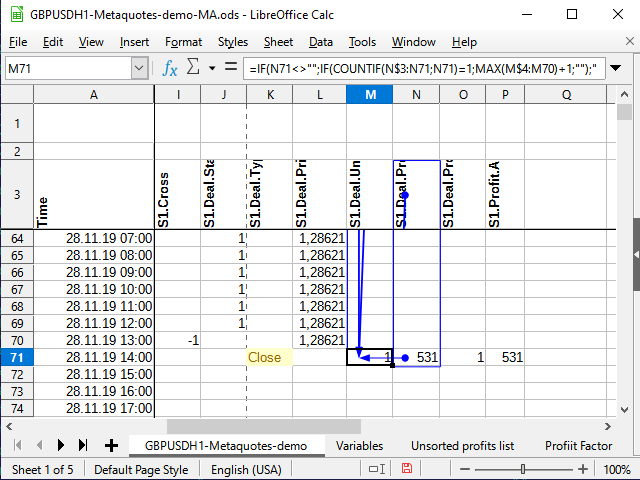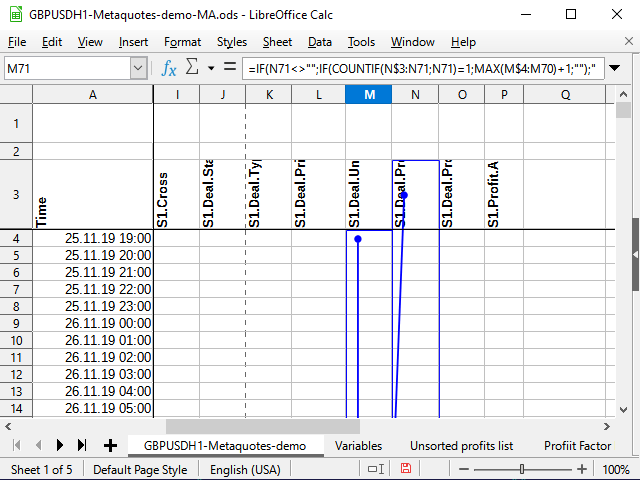`=IF(AND(N(N4)>0;I3=-1); 1 ;IF(AND(N(N4)<0;I3=-1); -1 ;IF(AND(N(N4)>0;I3=1); 2 ;IF(AND(N(N4)<0;I3=1); -2 ;""))))`

“module”只是不考虑符号的损益金额。它描述了在交易结束信号到来之前，价格在一个方向上走了多远。这可以帮助您选择止损和获利（即使它们不是原始策略所需要的）。

“module”公式非常初级，设置在P4中：
`=IF(N4<>"";ABS(N4);"")`

`=VLOOKUP( ROW(1:1);'GBPUSDH1-Metaquotes-demo'.\$M\$3:\$N\$6038; 2 )`

Vlookup范围的第一列（第二个参数）中查找某个值（第一个参数），然后返回位于相同检测行中的值，尽管在第三个参数中指定的列中（在我们的示例中，这是指定范围的第2列）。换句话说，从N列（从1开始）复制所有编号（唯一）的数值。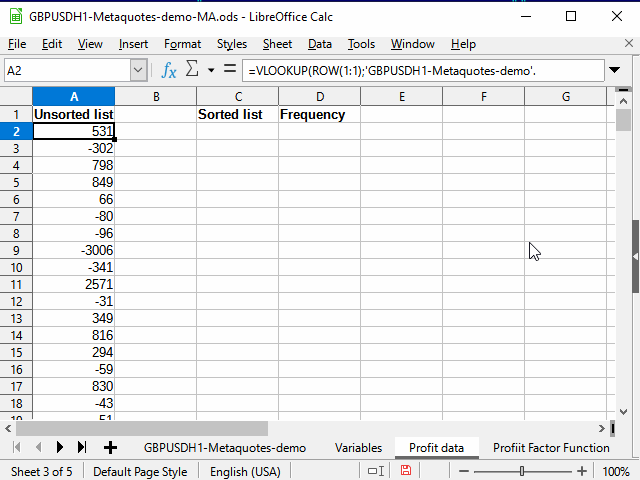`=COUNTIF('GBPUSDH1-Metaquotes-demo'.\$N\$4:\$N\$6038;C2)/COUNT('GBPUSDH1-Metaquotes-demo'.\$N\$4:'GBPUSDH1-Metaquotes-demo'.\$N\$6038)`

Count函数计算区间中的数值个数，Countif如果满足条件则执行相同的操作（在这种情况下，仅计算值等于C列单元格中的值的单元格）。

`=(MAX(\$'Profit data'.C2:\$'Profit data'.C214)-MIN(\$'Profit data'.C2:\$'Profit data'.C214))/(1+3,222*LOG10(COUNT('GBPUSDH1-Metaquotes-demo'.\$N\$4:'GBPUSDH1-Metaquotes-demo'.\$N\$6038)))`

`=C2`

`=F2+Interval`

G2单元格包含以下公式：

`=COUNTIFS('GBPUSDH1-Metaquotes-demo'.\$N\$4:\$N\$6038;">="&F2;'GBPUSDH1-Metaquotes-demo'.\$N\$4:\$N\$6038;"<"&F3)/COUNT('GBPUSDH1-Metaquotes-demo'.\$N\$4:\$N\$6038)`

CountifS（与Countif不同）允许接受多个条件，并将它们与“AND”运算符组合。其余的都一样。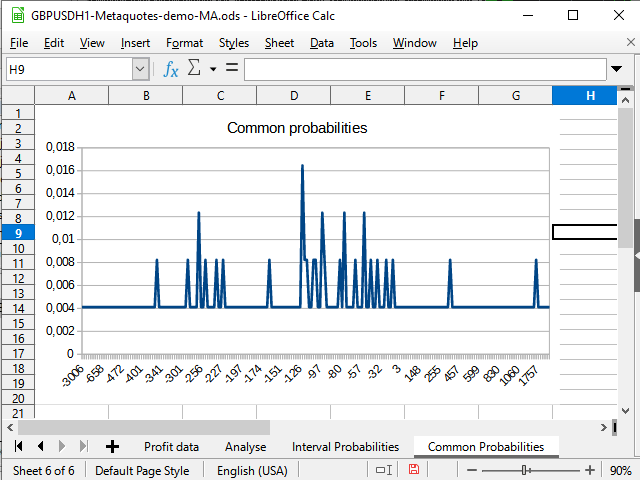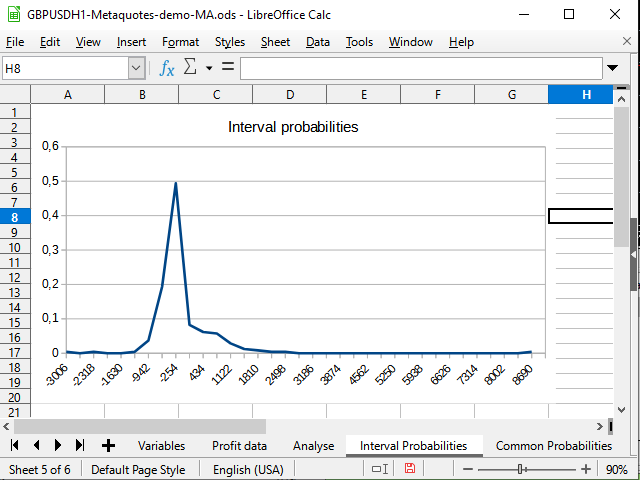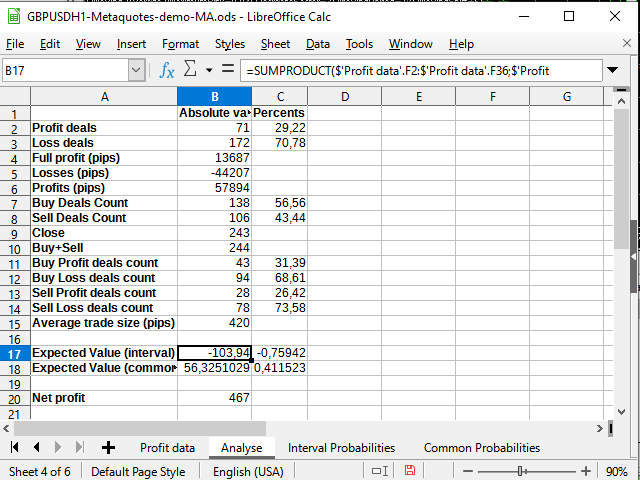### 在测试器中运行策略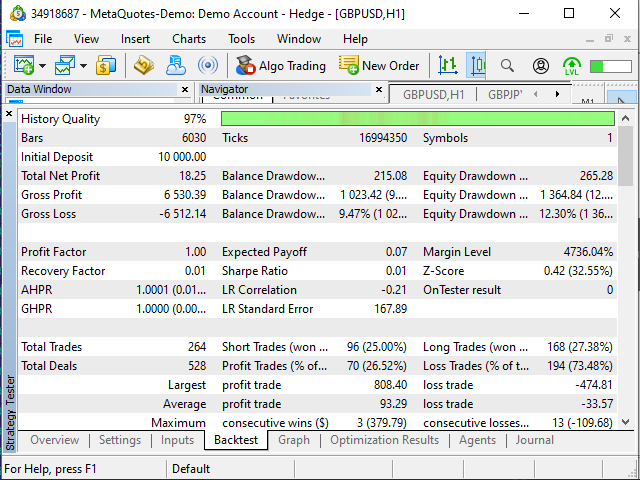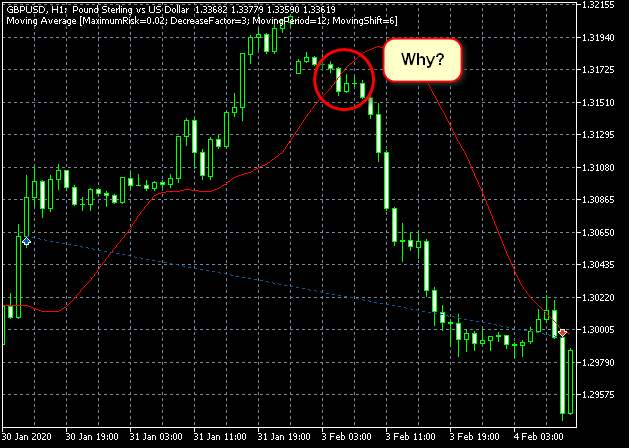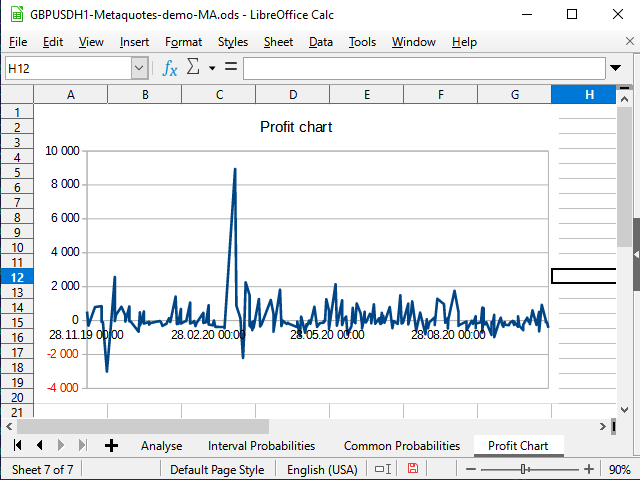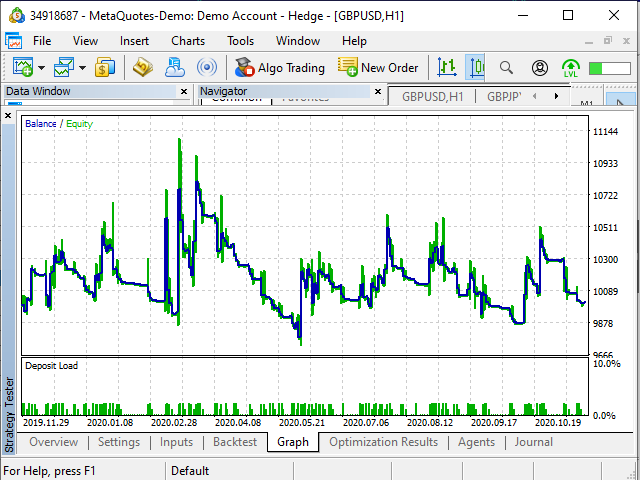### 结论

#### 该作者的其他文章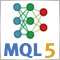神经网络变得轻松（第八部分）：关注机制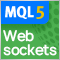MetaTrader 5 中的 WebSockets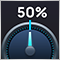开发自适应算法（第一部分）：寻找基本模式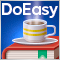DoEasy 函数库中的时间序列（第五十七·部分）：指标缓冲区数据对象